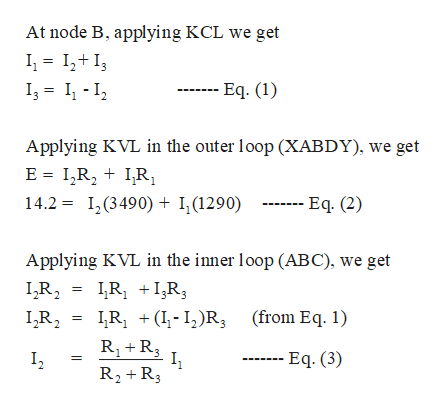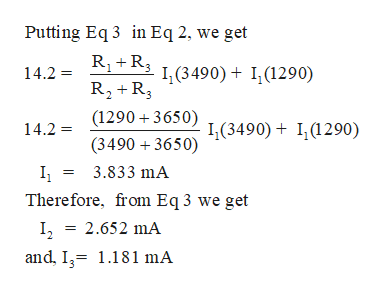Question
48 views
In the figure ε = 14.2 V, R1 = 1290 Ω, R2 = 3490 Ω, and R3 = 3650 Ω. What are the potential differences (in V) (a)  VA - VB, (b)  VB - VC, (c)  VC - VD, and (d)  VA - VC?
check_circle

Step 1

By symmetry, in the circuit, we can say that the current in the equals resistance are the same. In R1  there are a current of I1 , in R2 there are a current of I2 and in R3  there are I3.

Step 2

Now, from the circuit we can find the currents :help_outlineImage TranscriptioncloseAt node B, applying KCL we get I I -I2 --- Eq. (1) Applying KVL in the outer loop (XABDY), we get E I,R2 I,R, 14.2 I,(3490) + I,(1290) --- Eq. (2) Applying KVL in the imer loop (ABC), we get LR2 R IR RI R R1 R 3 (from Eq. 1) ---- Eq. (3) R2 R3 fullscreen
Step 3

Further,...help_outlineImage TranscriptionclosePutting Eq 3 in Eq 2, we get 14.2 1,(3490)+ I,(1290) R2 R3 (1290 3650) ( 3490) + I,(1290) 14.2 (34903650) I 3.833 mA Therefore, from Eq 3 we get I, = 2.652 mA and, I 1.181 mA fullscreen

### Want to see the full answer?

See Solution

#### Want to see this answer and more?

Solutions are written by subject experts who are available 24/7. Questions are typically answered within 1 hour.*

See Solution
*Response times may vary by subject and question.
Tagged in

### Current Electricity